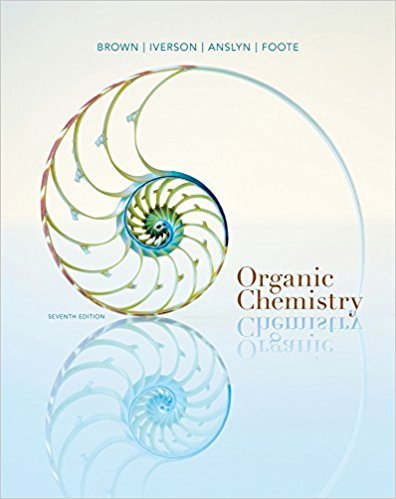×
Get Full Access to Organic Chemistry - 7 Edition - Chapter 13 - Problem 13.17
Get Full Access to Organic Chemistry - 7 Edition - Chapter 13 - Problem 13.17

×

# Following are 1H-NMR spectra for compounds G, H, and I, each with the molecularformulaISBN: 9781133952848 483

## Solution for problem 13.17 Chapter 13

Organic Chemistry | 7th Edition

• Textbook Solutions
• 2901 Step-by-step solutions solved by professors and subject experts
• Get 24/7 help from StudySoup virtual teaching assistantsOrganic Chemistry | 7th Edition

4 5 1 353 Reviews
25
2
Problem 13.17

Following are 1H-NMR spectra for compounds G, H, and I, each with the molecularformula C5H12O. Each is a liquid at room temperature, is slightly soluble in water, andreacts with sodium metal with the evolution of a gas.(a) Propose structural formulas of compounds G, H, and I.(b) Explain why there are four lines between d 0.86 and 0.90 for compound G.(c) Explain why the 2H multiplets at d 1.5 and 3.5 for compound H are so complex.

Step-by-Step Solution:
Step 1 of 3

Chapter – 4 Convective Mass Transfer 4.1 Introduction 4.2 Convective Mass Transfer coefficient 4.3 Significant parameters in convective mass transfer 4.4 The application of dimensional analysis to Mass Transfer 4.4.1 Transfer into a stream flowing under forced convection 4.4.2 Transfer into a phase whose motion is due to natural convection 4.5 Analogies among mass, heat, and momentum transfer 4.5.1 Reynolds analogy 4.5.2 Chilton – Colburn analogy 4.6 Convective mass transfer correlations 4.6.1 For flow around flat plat 4.6.2 For flow around single sphere 4.6.3 For flow around single cylinder 4.6.4 For flow through pipes 4.7 Mass transfer between phases 4.8 Simultaneous heat and mass transfer 4.8.1 Condensation of vapour on cold surface 4.8.2 Wet bulb thermometer 4.1 Introduction Our discussion of mass transfer in the previous chapter was limited to molecular diffusion, which is a process resulting from a concentration gradient. In system involving liquids or gases, however, it is very difficult to eliminate convection from the overall mass­transfer process. Mass transfer by convection involves the transport of material between a boundary surface (such as solid or liquid surface) and a moving fluid or between two relatively immiscible, moving fluids. There are two different cases of convective mass transfer: 1. Mass transfer takes place only in a single phase either to or from a phase boundary, as in sublimation of naphthalene (solid form) into the moving air.

Step 2 of 3

Step 3 of 3

##### ISBN: 9781133952848

The answer to “Following are 1H-NMR spectra for compounds G, H, and I, each with the molecularformula C5H12O. Each is a liquid at room temperature, is slightly soluble in water, andreacts with sodium metal with the evolution of a gas.(a) Propose structural formulas of compounds G, H, and I.(b) Explain why there are four lines between d 0.86 and 0.90 for compound G.(c) Explain why the 2H multiplets at d 1.5 and 3.5 for compound H are so complex.” is broken down into a number of easy to follow steps, and 76 words. This textbook survival guide was created for the textbook: Organic Chemistry, edition: 7. Organic Chemistry was written by and is associated to the ISBN: 9781133952848. This full solution covers the following key subjects: . This expansive textbook survival guide covers 24 chapters, and 1131 solutions. Since the solution to 13.17 from 13 chapter was answered, more than 241 students have viewed the full step-by-step answer. The full step-by-step solution to problem: 13.17 from chapter: 13 was answered by , our top Chemistry solution expert on 03/16/18, 05:02PM.

Unlock Textbook Solution